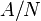# Homomorphism set is disjoint union of injective homomorphism sets

## Statement

### For groups

Suppose$A$ and$B$ are groups. Then, the set of homomorphisms$\operatorname{Hom}(A,B)$ can be identified with the disjoint union of the sets of injective homomorphisms from$A/N$ to$B$ for every normal subgroup$N$, i.e.,:$\operatorname{Hom}(A,B) \cong \bigsqcup_{N \underline{\triangleleft} A} \operatorname{IHom}(A/N,B)$

where$\operatorname{IHom}$ denotes the set of injective homomorphisms. Here$\cong$ denotes a canonical bijection of the sets.

The correspondence is as follows:

• For any homomorphism from$A$ to$B$, we denote its kernel by$N$. By the first isomorphism theorem, there is an isomorphism$A/N$ to the image of the homomorphism, which when composed with the inclusion map to$B$, gives an injective homomorphism from$A$ to$B$.
• Conversely, given an injective homomorphism$A/N$ to$B$, we compose with the quotient map$A \to A/N$ to get a homomoorphism from$A$ to$B$ with kernel precisely$N$.